1. Home
2. Blog

# Calculation of displacement value in easy way

by egpat       02 Mar 2020

How we make suppositories? Simply we mix drug with suppository base and pour into the moulds of one or two gram to bring the dosage form. Is that so simple? How much base we should use? That’s the point to consider. As the drug and suppository base may have different densities, they would occupy different volumes under equal weights. So 1 gm of drug may not displace 1 gm of base exactly and we need to assess how much base is to be displaced by the presence of the drug.

To understand this well, let’s go with a simple example.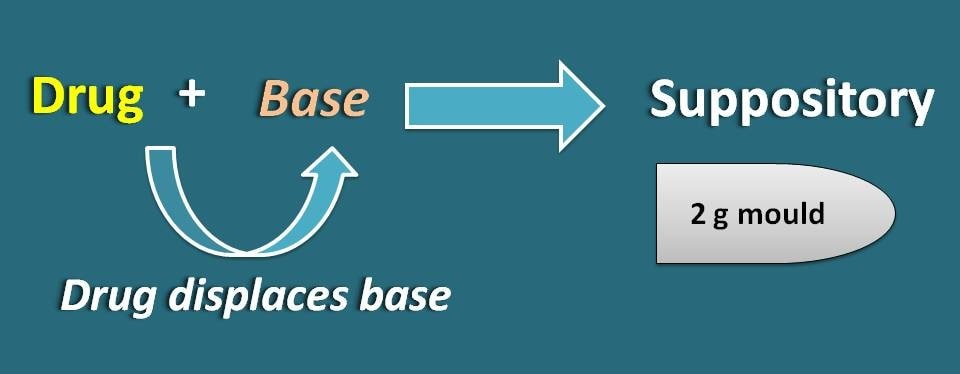## What is Displacement value?

Let’s consider that we have six empty suppository moulds each of 2 gm capacity. Now if we fill these suppositories with only base without any drug, then the total weight of the base required for filling will be equal to 6 x 2=12 gm. This is considered as theoretical weigh of the suppository base.Now let see what happens practically. When these six suppositories are filled with both suppository base and drug, the total weight of these six suppositories may not be equal to 12 grams as some part of the base is displaced by drug due to difference in the densities.

Suppose we have prepared six suppositories such that 500 mg of the drug is present in each suppository and we find practical weight of these suppositories, suppose, as 14 gm. Now the practical weight of the six suppositories is 14 gm and the total amount of the drug in all suppositories is equal to 6 * 500=3000 mg=3 g.

Practical weight=14 gm

Drug weight=3 gm

So, the weight of the suppository base is 14 – 3=11 gm.

So, by the addition of the drug , 1 gm of the suppository base is displaced.

## Theoretical and practical weights

We can also calculate displacement value in another way. Let’s consider the previous example.

Theoretical weigh of base=12 gm

Theoretical weight of drug=3 gm

Theoretical weight of base + drug=12 + 3=15 gm

But we found the practical weight of six suppositories as 14 gm. So the difference will be equal to 15-14=1gm.So, 1gm of the base is displaced by 3 gm of the drug. This is nothing but displacement value of the drug.

Now we can define displacement value as follows.

It is the amount of the drug that displaces 1 gm of the suppository base.

Now in the following section, let’s discuss how can we calculate the displacement value along with few examples.

## Practical example 1:

Calculate the displacement value of drug If a prescription requires 400 mg of drug per suppository weighing 2 gm. The weight of 6 suppositories with required drug weigh 13.8 g?

Solution:

Let’s see what the data given here.

No. of suppositories (n)=6

Total weight of suppositories (W)=13.8 gm

Weight of Drug (d)=400 mg

Now, we have to calculate displacement value. First let's calculate theoretical weight.

Calculation of theoretical weight

Weight of Base (B)=6 x 2=12 g

Weight of Drug (D)=400 mg x 6=2400 mg=2.4 g

Theoretical weight=12 + 2.4=14.4 g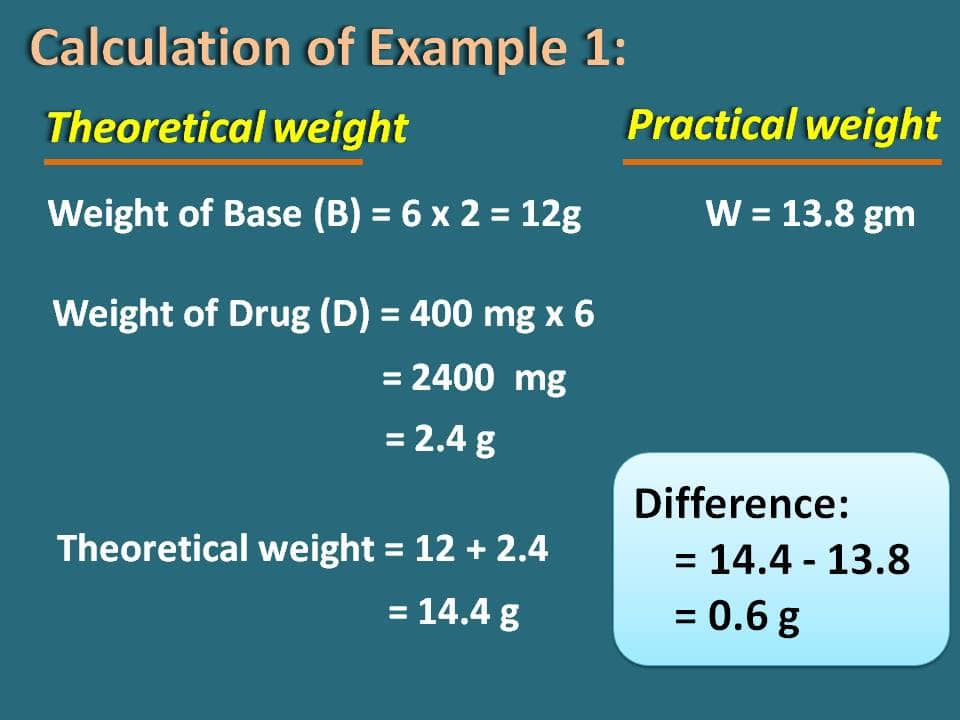Now practical weight is given as 13.8 gm, therefore the difference is 14.4 – 13.8=0.6 gm

In another words, 0.6 gm of the base is displaced by 2.4 gm of drug. So 1 gm of the base is displaced by (2.4/0.6) x 1=4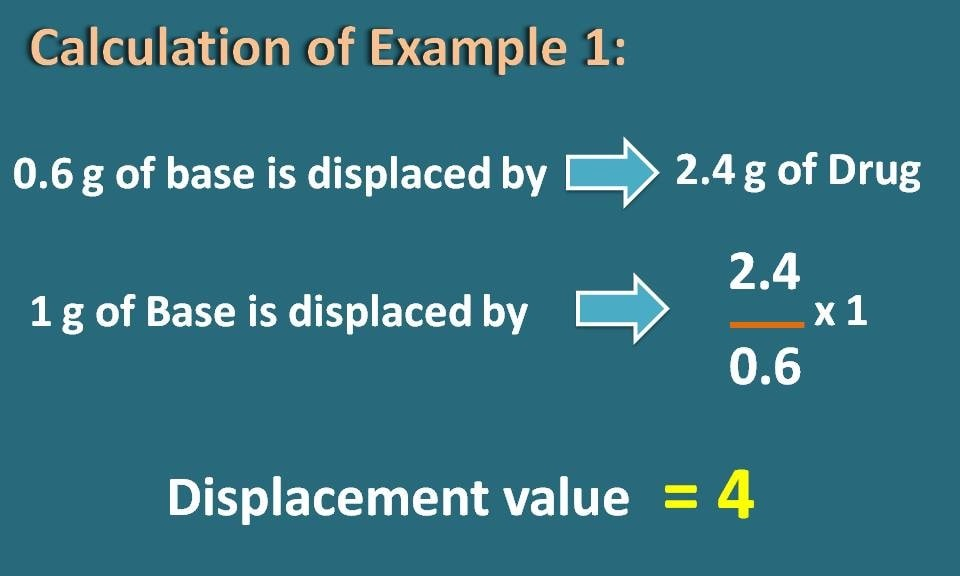### Easy calculation by using formula:

We can use the following formula to calculate the displacement value directly.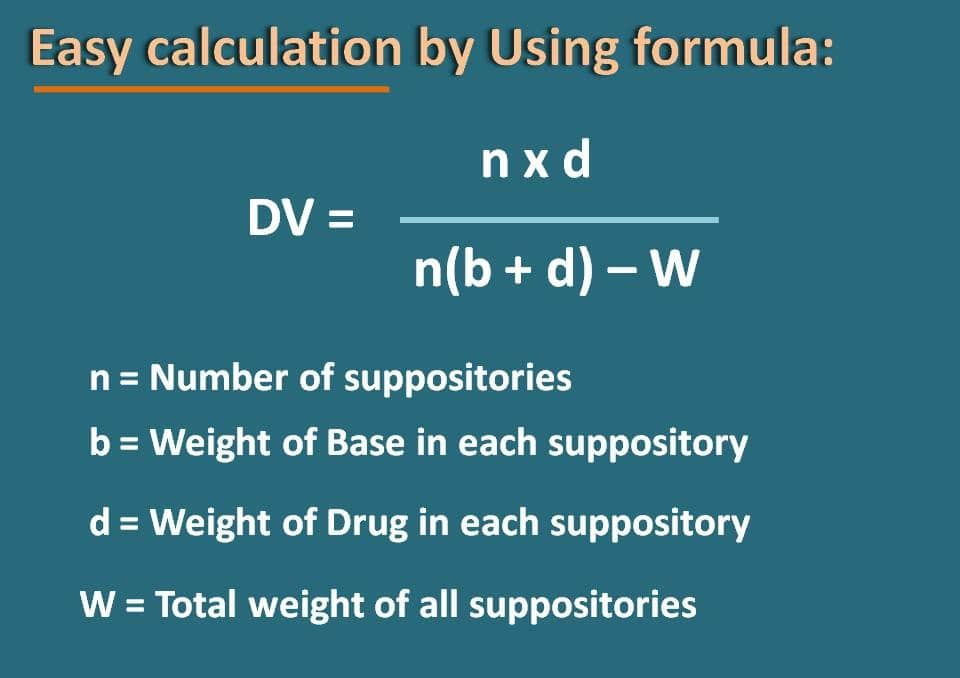Here b and d indicate the theoretical weight of the base and drug respectively in each suppository. The term W indicates the practical weight of all the suppositories filled with base and drug.

Now, let’s apply the above formula to calculate the displacement value.

Displacement value=2.4 / (12 + 2.4 -13.6)=4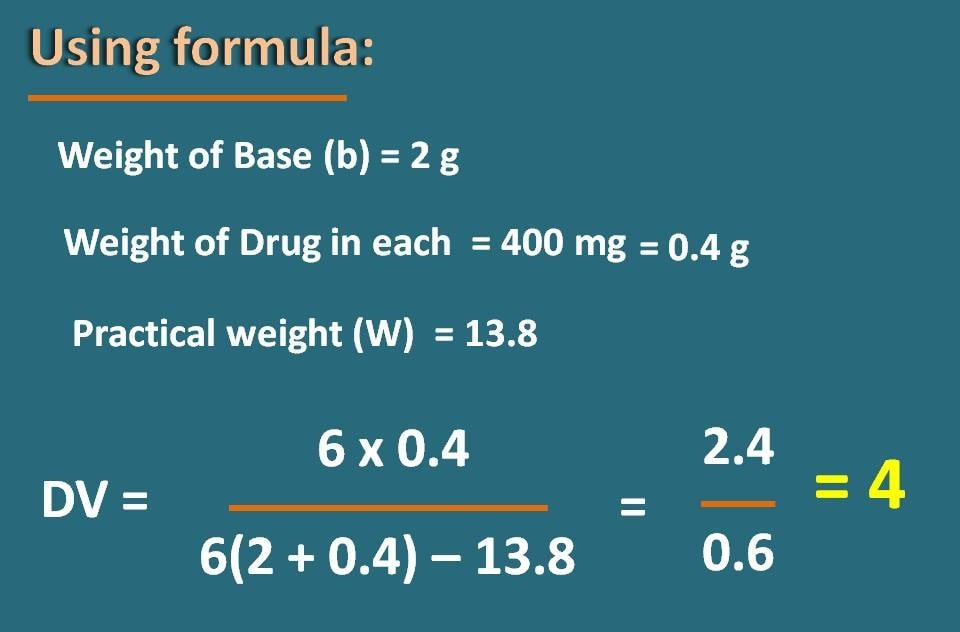So this is a simple formula that can be easily remembered to calculate the displacement value of the drug in suppository in an easy way.

Now let’s go with another example where the amount of the drug in each suppository is expressed in terms of percentage.

## Practical example 2:

If 12 cocoa butter suppositories containing 40% of drug weigh 15.5 grams, what is the displacement value of drug?

Note: Suppository is made up of 1-g mould.

Solution:

Again let’s see what the data given here.

No. of suppositories (n)=12

Total weight of suppositories (W)=15.5 gm

Weight of Drug (D)=40%

Now, we have to calculate displacement value.

Calculation of theoretical weight

Weight of Base (B)=12 x 1=12 g

Here the drug in each suppository is 40% so the total concentration of drug in all six suppositories will be again 40%. If we multiply this concentration with total weight of the suppositories, we will get the total weight of the drug in all suppositories.

Weight of Drug (D)=40% x 15.5=0.4 x 15.5=6.2 g

Theoretical weight=12 + 6.2=18.2 gNow practical weight is given as 15.5 gm, therefore the difference is 18.2 – 15.5=2.7 gm

In another words, 2.7 gm of the base is displaced by 6.2 gm of drug. So 1 gm of the base is displaced by (6.2/2.7) x 1=2.3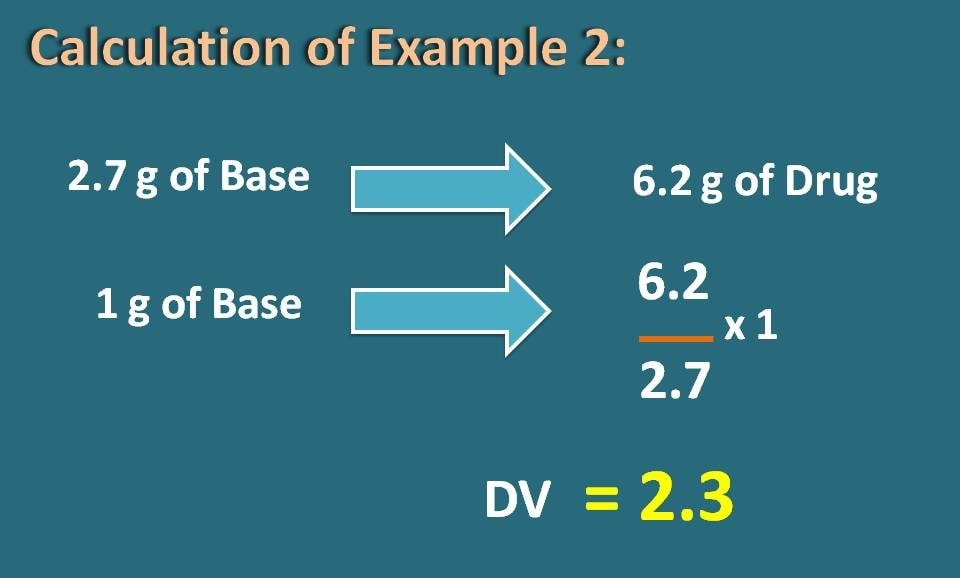### Easy calculation by using formula:

Now we can modify the formula slightly as the concentration of drug is given in terms of percentage.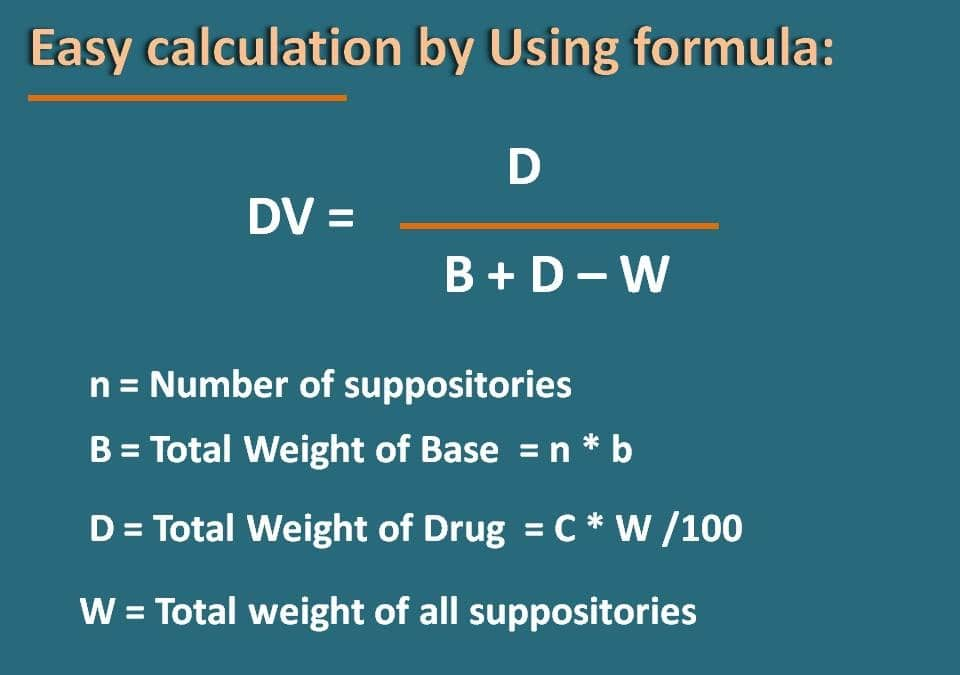Here B and D indicate the total theoretical weight of the base and drug respectively in all suppositories. The term W indicates the practical weight of all the suppositories filled with base and drug.

Now, let’s apply the above formula to calculate the displacement value.

Displacement value=6.2 / (12 + 6.2 -15.5)=2.3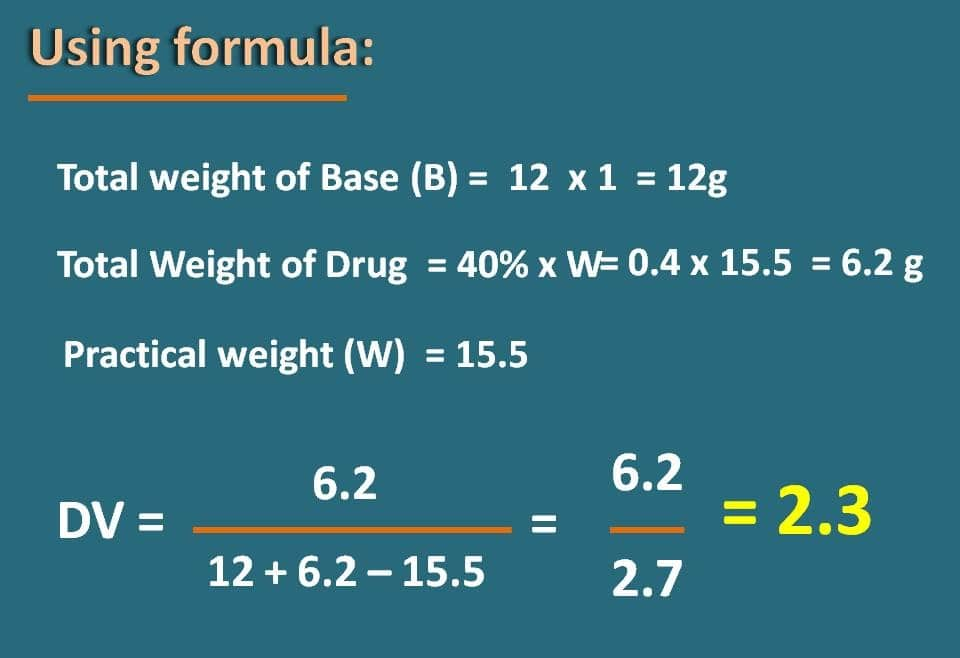Here is a video on how to calculate displacement value in case of suppositories.

## Conclusion

In this way, displacement value is an essential parameter that is to be calculated before formulating the suppository and it gives the estimation the base that is needed to prepare a suppository with require weight of the drug. It can be easily calculated by using simple formula where it directly gives the final value with simple calculation without any confusion.

Hope you enjoyed the reading and don’t forget to share this post if you like it. Have a nice day!